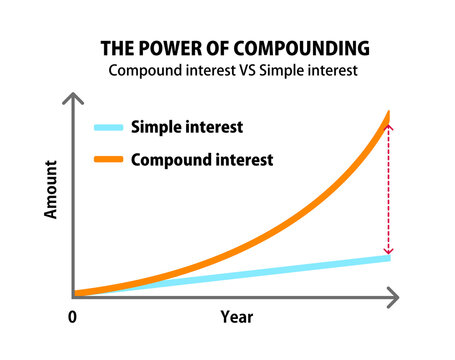# Interest Rates

The interest rate is the amount charged on top of the principal by a lender to a borrower for the use of assets and it also applies to the amount earned at a bank or credit union from a deposit account.Banks borrow money from you in the form of deposits, and interest is what they pay you for the use of the money deposited. They use the money from deposits to fund loans.

For Example, If a person borrows a loan of 300000 from a bank with an interest of 3% p.a for a period of 5 years. So, the interest calculated on the principal will be of 45000.Here, the above calculation was on simple interest and there’s another type of interest called compound interest.

## Different Types of Interest Rates

#### Based on Time

• Simple Interest: This interest type is known as simple because of its name as the as it calculated on the principal as for the period of holding. It is Calculated as P X R X T.• Compound Interest: this interest means that the borrower pays even more in interest. Compound interest, also called interest on interest, is applied to the principal but also on the accumulated interest of previous periods.

It is Calculated as p X [(1 + interest rate)n − 1].In the case of the above example, the interest will be 47782.223 which is more than the simple interest.• Continuously compounded interest: It is the mathematical limit of the general compound interest formula, with the interest compounded an infinitely many times each year. It is calculated as P * [(eR*T) – 1].

#### Based on Variability

• Fixed rates remain the same throughout the life of the loan. Initially, your payments consist mostly of interest payments. As time goes on, you pay a higher and higher percentage of the debt principal. Most conventional mortgages are fixed-rate loans.
• Variable rates change with the prime rate. When the rate rises, so will the payment on your loan. With these loans, you must pay attention to the prime rate, which is based on the fed funds rate. With either type of loan, you can generally make an extra payment at any time toward the principal, helping you to pay the debt off sooner.#### Based on Level

• High-interest rates make loans more expensive. When interest rates are high, fewer people and businesses can afford to borrow. That lowers the amount of credit available to fund purchases, slowing consumer demand. At the same time, it encourages more people to save because they receive more on their savings rate. High-interest rates also reduce the capital available to expand businesses, strangling supply. This reduction in liquidity slows the economy.
• Low-interest rates have the opposite effect on the economy. Low mortgage rates have the same effect as lower housing prices, stimulating demand for real estate. Savings rates fall. When savers find they get less interest on their deposits, they might decide to spend more. They might also put their money into slightly riskier but more profitable investments, which drives up stock prices.

### Conclusion

The interest rates are an integral parts of the economy and banking system of any country. It manages most of its debt repayment and also helps in increasing the revenues for it.